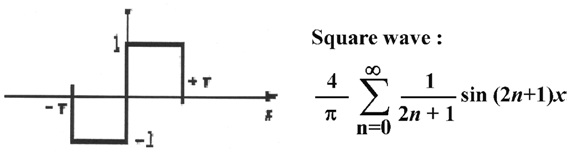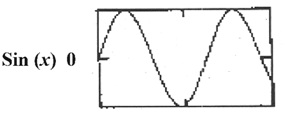• www.uptuexam.com
• GBTU Results 2014
• MTU Results 2014
• UPTU Circulars
• COP Result 2012,13,14
• UPTU Previous Papers
• GATE study material
• Robotics Projects
Thank you for visiting www.UPTUexam.com " Free download Exam materials, previous year question papers, free ebooks & Providing Important Questions To Do their Exams Well and Totally a FREE SERVICE for all engineering streams (B.Tech)."

### Communication Lab – II

# EXPERIMENT No. :  01

Aim: To construct a triangular wave with the help of fundamental frequency and its harmonic component.
Apparatus Required:

 S. No. Component Quantity 1. Trainer kit ST2603 1 2. CRO 1 3. Connecting leads 2

Theory:  Fourier synthesis is a method of electronically constructing a signal with a specific, desired periodic waveform. It works by combining a sine wave signal and sine wave or cosine-wave harmonics (signals at multiples of the lowest, or fundamental, frequency) in certain proportions. The scheme gets its name from a French mathematician and physicist named Jean Baptiste Joseph Baron de Fourier, who

# lived during the 18th and 19th centuries.

A mathematical theorem stating that a Periodic function f(x) which is reasonably continuous may be expressed as the sum of a series of sine or cosine terms (called the Fourier series), each of which has specific Amplitude and Phase coefficients known as Fourier coefficients.Many waveforms represent signal energy at a fundamental frequency and also at harmonic frequencies (whole-number multiples of the fundamental). The relative proportions of energy concentrated at the fundamental and harmonic frequencies determine the shape of the wave. The wave function (usually amplitude, frequency, or
phase versus time) can be expressed as of a sum of sine and cosine functions called a Fourier series, uniquely defined by constants known as Fourier coefficients. If these coefficients are represented by a0, a1, a2, a3, ..., an, ... and b1, b2, b3, ..., bn ..., then the Fourier series F(x), where x is an independent variable (usually time), has the following form :

F(x) = a0/2 + a1 cos x + b1sin x + a2 cos 2x + b2 sin 2x + ... + an cos nx + bn sin nx +...

In Fourier synthesis, it is necessary to know, or to determine, the coefficients a0, a1 a2, a3, ..., an ... and b1 b2, b3, ..., bn ... that will produce the waveform desired when "plugged into" the generalized formula for the Fourier series. Then, sine and cosine waves with the proper amplitudes (as defined by the coefficients)
must be electronically generated and combined, up to the highest possible value of n. The larger the value of n for which sine-wave and cosine-wave signals are generated, the more nearly the synthesized waveform matches the desired waveform.

Following is the fourier series equation and wave form for the triangular wave.i.e equation for triangular wave is
A*( cos x + 1/9 cos 3x + 1/25 cos 5x + ………)
where A = 8/()2
Example:- (0V DC + 3Cos(x) + 0.44 Cos (3x) + 0.304 Cos(5x) + 0.152 Cos(7x) + 0.092 Cos(9x))

Procedure :
1. Switch ‘On’ the Power Switch and LCD.
2. Minimize all the amplitudes of frequencies by potentiometer.
3. Set DC potentiometer to 0V DC by using Output BNC & Oscilloscope DC mode.
4. Switch each frequency for cosine and positive value.
5. Use “Harmonic Output” BNC to set and observe individual harmonic and use “Output” BNC to observe the resultant waveform.
6. Use Harmonic select push button switch and Set the fundamental cosine Frequency (1 KHz) to 3V, observe it on oscilloscope.
7. Use Harmonic select push button switch and Set the 3rd harmonics i.e. cos 3x to 0.44V.
8. Use Harmonic select push button switch and Set the 5th harmonics i.e. cos 5x to 0.30V, 7th harmonic i.e. cos7x to 0.16V & 9th harmonic i.e. cos9x to 0.08VObservation:
Result: Traced the waveform from CRO & observed the effect of harmonics on the waveform.
Precautions:
·         Make sure that kit is powered off when connections are made.
·         Handle the trainer kit properly.
Pre Expeiment Questions:
Q:1 What is Fourier Synthesis?
Q:2 What is the harmonics of the Fourier Series?
Q:3 The effect of various harmonics on the waveform?

Post Experiment Questions:

//////////////////////////////////////////////////////////////////////////////////////////////////////////////////////////////////////////////////////////

# EXPERIMENT No. :  02

Aim: To construct a square wave with the help of fundamental Frequency and its harmonic component.

Apparatus Required:

 S. No. Component Quantity 1. Trainer kit ST2603 1 2. CRO 1 3. Connecting leads 2

Theory:  Fourier synthesis is a method of electronically constructing a signal with a specific, desired periodic waveform. It works by combining a sine wave signal and sine wave or cosine-wave harmonics (signals at multiples of the lowest, or fundamental, frequency) in certain proportions. The scheme gets its name from a French mathematician and physicist named Jean Baptiste Joseph Baron de Fourier, who

# lived during the 18th and 19th centuries.

A mathematical theorem stating that a Periodic function f(x) which is reasonably continuous may be expressed as the sum of a series of sine or cosine terms (called the Fourier series), each of which has specific Amplitude and Phase coefficients known as Fourier coefficients.Many waveforms represent signal energy at a fundamental frequency and also at harmonic frequencies (whole-number multiples of the fundamental). The relative proportions of energy concentrated at the fundamental and harmonic frequencies determine the shape of the wave. The wave function (usually amplitude, frequency, or
phase versus time) can be expressed as of a sum of sine and cosine functions called a Fourier series, uniquely defined by constants known as Fourier coefficients. If these coefficients are represented by a0, a1, a2, a3, ..., an, ... and b1, b2, b3, ..., bn ..., then the Fourier series F(x), where x is an independent variable (usually time), has the following form :

F(x) = a0/2 + a1 cos x + b1sin x + a2 cos 2x + b2 sin 2x + ... + an cos nx + bn sin nx +...

In Fourier synthesis, it is necessary to know, or to determine, the coefficients a0, a1 a2, a3, ..., an ... and b1 b2, b3, ..., bn ... that will produce the waveform desired when "plugged into" the generalized formula for the Fourier series. Then, sine and cosine waves with the proper amplitudes (as defined by the coefficients)
must be electronically generated and combined, up to the highest possible value of n. The larger the value of n for which sine-wave and cosine-wave signals are generated, the more nearly the synthesized waveform matches the desired waveform.

Following is the fourier series equation and wave form for the square wave.i.e. equation for rectangular sawtooth wave is
A *(sin x + 1/3 sin3x + 1/5sin5x)
where A = 4/
Example:- 0V DC + 5 Sin(x) + 1.72 Sin(3x) + 1.04 Sin(5x) + 0.74 Sin(7x) + 0.56 Sin(9x)

Procedure :
1. Switch ‘On’ the Power and LCD Switch.
2. Minimize all the amplitudes of frequencies by potentiometer.
3. Set DC potentiometer to 0V DC by using Output BNC & Oscilloscope DC mode.
4. Switch each odd frequency for sine and positive value.
5. Use “Harmonic Output” BNC to set and observe individual harmonic and use “Output” BNC to observe the resultant waveform.
6. Use Harmonic select push button switch and set the fundamental sine Frequency H1 (1 KHz) to 5V observe it on oscilloscope.7. Use Harmonic select push button switch and set the 3rd harmonics H3 i.e. sin3x to 1.72V output should be similar to following waveform.
8. Use Harmonic select push button switch and set the harmonic H5 (sin5x) to 1.04V and observe the output.
9. Use Harmonic select push button switch and set the harmonic H7 (sin7x) to 0.74V and observe the output.
10. Use Harmonic select push button switch and set the harmonic H9 (sin9x) to 0.56V and observe the output.Observation:
Result: Traced the waveform from CRO & observed the effect of harmonics on the waveform.
Precautions:
·         Make sure that kit is powered off when connections are made.
·         Handle the trainer kit properly.

Pre Expeiment Questions:
Q:1 What is Fourier Synthesis?
Q:2 What is the harmonics of the Fourier Series?
Q:3 The effect of various harmonics on the waveform?